### Plotting and visualization with python using matplotlib

Course content:

• A brief matplotlib API primer:

1. Colors, Markers, and Line styles.

2. Figures and Subplots.

3. Ticks, labels, legends, and saving plots to file.

• Different plots using matplotlib, pandas, and seaborn:

1. Line & bar plots.

2. Histograms & density plots.

3. Scatter or point plots.

4. Facet grids and categorical data.

Overview:

• Making informative visualizations (sometimes called plots) is one of
the most important tasks in data analysis. It may be a part of the
exploratory process—for example, to help identify outliers or needed
data transformations, or as a way of generating ideas for models.

• Python has many add-on libraries for making static or dynamic
visualizations, we will mainly be focused on matplotlib and libraries
that build on top of it. matplotlib is a desktop plotting package
designed for creating (mostly two dimensional) publication-quality
plots.

• The project was started by John Hunter in 2002 to enable MATLAB-like
plotting interface in Python.

• Over time, matplotlib has spawned a number of add-on toolkits for data
visualization that uses matplotlib for their underlying plotting. One of
these is seaborn, which we will explore later in this
presentation.

A brief matplotlib API Primer:

• With matplotlib, we use the following import convention.

• After running it in jupyter, we can try creating a simple plot.If
everything is set up right, a line plot like below appear.

`  import pandas as pdimport numpy as npimport matplotlib.pyplot as pltdata=np.array([2,6,4,8,3,4])plt.plot(data)`
`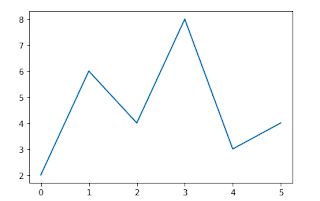`

Figures and subplots:

• Plots in matplotlib reside within a Figure object. You can create a
new figure with pit.figure().

• plt.figure() has a number of options; notably, figsize will guarantee
the figure has a certain size and aspect ratio if saved to disk. One
can’t make a plot with a blank figure.One have to create one or more

• This means that the figure should be 2 x2 (so up to four plots in
total), and we’re selecting the first of four subplots (numbered from
1).

• The objects returned by fig.add_subplot are Axessubplot objects, on
which one can directly plot on the other empty subplots by calling
each one's instance method.

`fig=plt.figure()ax1=fig.add_subplot(2,2,1)ax2=fig.add_subplot(2,2,2)ax3=fig.add_subplot(2,2,3)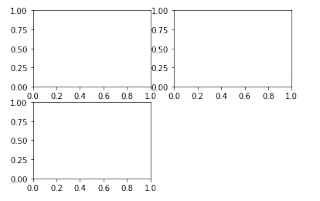x=np.arange(0,3*np.pi,0.1)fig=plt.figure()ax1=fig.add_subplot(2,2,1)ax2=fig.add_subplot(2,2,2)ax3=fig.add_subplot(2,2,3)ax1.plot(np.random.randn(10))ax2.plot(np.sin(x))ax3.plot(np.cos(x))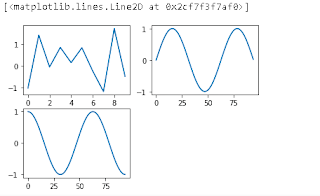fig,axes=plt.subplots(2,2)axes`
`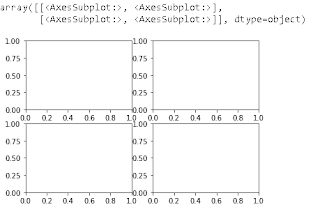`

Colors,Markers,and line styles:

• Matplotlib’s main plot function accepts arrays of x and y coordinates
and
optionallyastring abbreviation indicating color and line style.

• There are a number of color abbreviations provided for commonly used
colors, but one can use any color on the spectrum by specifying its hex
code (e.g.,'#CECECE’).

• One can see the full set of line styles by looking at the command
help(pit.plot).

• Line plots can additionally have markers to highlight the actual data
points.

`  help(plt.plot)Output=Help on function plot in module matplotlib.pyplot:plot(*args, scalex=True, scaley=True, data=None, **kwargs)    Plot y versus x as lines and/or markers.        Call signatures::            plot([x], y, [fmt], *, data=None, **kwargs)        plot([x], y, [fmt], [x2], y2, [fmt2], ..., **kwargs)        The coordinates of the points or line nodes are given by *x*, *y*.     data=np.random.randn(30)dataOutput=array([-0.55620921,  1.15354551,  1.88428619, -0.25361657,  0.11720929,        0.99380999, -1.61673998,  0.15259621,  1.07661434,  0.57335323,        1.51775676,  2.16336357,  0.29821075,  0.80887232, -0.23170136,       -0.15509185, -2.13596732, -0.25341181, -0.81853445, -1.75597032,       -2.66753714, -1.10821393,  1.40548605,  0.06021546, -0.4025957 ,       -0.18417526,  3.20519396, -1.15411711,  0.58264083, -0.070976  ])plt.plot(data,linestyle='--',color='g')`
`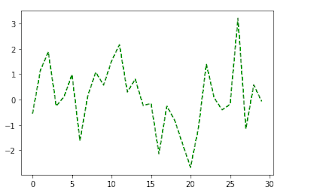`
`plt.plot(data,color='y',linestyle='dashed',marker='o') `

Ticks, Labels and Legends:

• The pyplot interface, designed for interactive use, consists of methods
like xlim, xticks, and xticklabels. These control the plot range, tick
locations, and tick labels respectively.

• To change the x-axis ticks, it’s easiest to use set_xticks and
set_xticklabels. The former instructs matplotlib where to place the
ticks along the data range; by default these locations will also be the
labels. The rotation option sets the x tick labels at a any degree
rotation.

• Lastly, set_xlabel gives a name to the x-axis 1 and set_title the
subplot title.

• Legends are another critical element for identifying plot elements. One
pass the label argument when adding each piece of the plot.

`fig=plt.figure()ax=fig.add_subplot(1,1,1)ax.plot(data)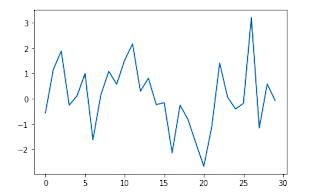fig=plt.figure()ax=fig.add_subplot(1,1,1)ax.plot(data)ax.set_xticks([0,10,20,30])ax.set_xticklabels(['zero','ten','twenty','thirty'],rotation=30,fontsize='large')ax.set_title("My plot")ax.set_xlabel("Stages")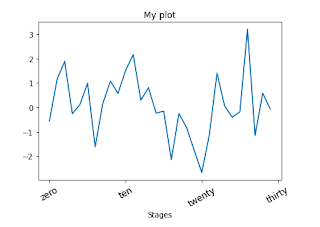fig=plt.figure()ax=fig.add_subplot(1,1,1)ax.plot(np.sin(x),'b--',label="Sine wave")ax.plot(np.cos(x),'c.',label="Cosine wave")ax.legend()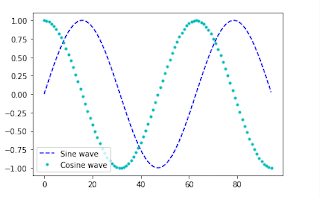`

Saving plots to file:

• One can save the active figure to file using plt.savefig. This method
is equivalent the figure object’s savefig instance method.

• For example, to save an PNG version of a figure, we need only type:
plt.savefig(‘image.png')

• The file type is inferred from the file extension. So if one used .pdf
instead, one would get a PDF.

• There are a couple of important options that are used frequently : dpi,
which controls the dots-per-inch resolution, and bbox_inches, which can
trim the whitespace around the actual figure.

`plt.plot(data,color='k',linestyle='dashed',marker='o')plt.savefig("image11.png")`

Different plots using matplotlib, pandas, and seaborn:

• Bar plot

• Histogram

• Scatter plot

• Stack plot

• Pie plot

`Plotting using Seabornimport seaborn as snssns.set()data=np.random.randn(30)sns.distplot(data,kde=0)`
`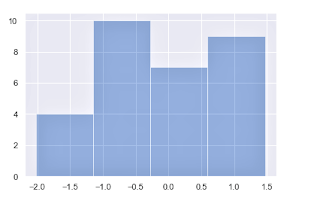`

I hope that you have understood this topic, I have tried my best, This is a vast topic but I have covered it in short....

Best Regards from,
msbtenote:)
THANK YOU!!!